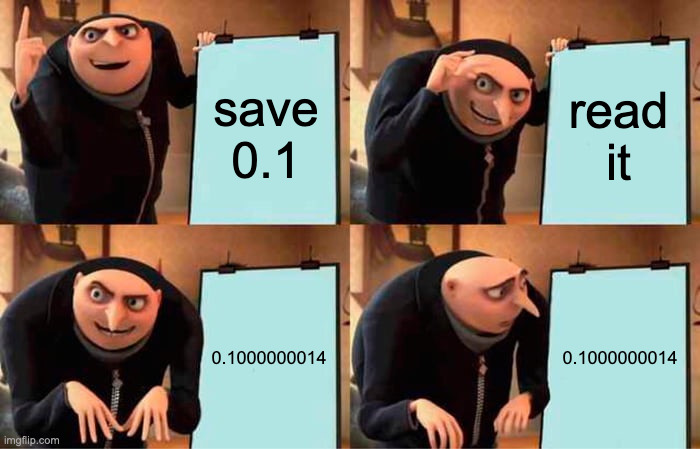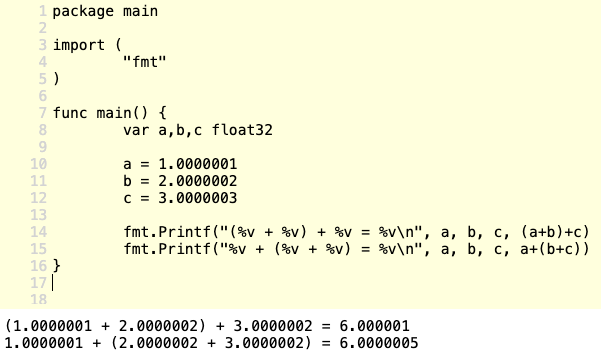We're building the future of Supply Chain Security. View Roles

## Floating point numbers, and why they suck## Introduction

Recently I was looking at some data I had just modelled in our data warehouse, to check everything looked correct, and found something weird: I saw some events for a value change where the previous and new values were equal.

Our calculation is smart; we compare the newly calculated value to the one we calculated previously and only save it if it actually changed, incrementing the version number as we do so.

I was seeing some of the stats we had in our warehouse had a very high version number. This implied many changes had happened, and this number was suspiciously big. Here's an example of one of the events:

``````{
"version": "933",
"modified_at": "2021-11-09 12:40:04.427087 UTC",
"new_values": {
"avg_compliance_percentage": "90.34326",
},
"prev_values": {
"avg_compliance_percentage": "90.34326",
}
},
``````

There were no changes and yet another version was being saved!

A quick double-check in our code reassured me we were requiring the previous and new values to be different to save it. This check was not being skipped anywhere! So what was going on?

## They were not Equal

I rushed to check the data in our production database, maybe this was an issue with how we were sending the data to the warehouse. I searched for the same stats and I found they were actually not identical. And they also had more decimal places.

``````{
"current_avg_compliance_percentage": "90.34326374227855",
"previous_avg_compliance_percentage": "90.34326374227852"
}
``````

There was a massive `0.00000000000003` difference right there in front of me. (3E-14)

So that's it? Problem solved? The versions are actually different, and we are just not outputting enough precision to our data warehouse?

Well yes, but actually no. We are sending the data to the warehouse as a float with 32 bit precision instead of double (64 bit) precision, so that would explain the data warehouse having fewer digits.

But I knew this specific value that was being calculated could not have changed by so little. In this context, the value is a compliance percentage and the smallest possible change depends on the number of suppliers that a client has.

``````minChange = 100/(numSuppliers * numControls)
``````

Because the number of active controls is a value we know (203 at the time of writing), a client would need to have at least `16,420,361,247,948` (16 trillion+) suppliers. Now, Risk Ledger's network is growing quickly, but I'm pretty sure we haven't got a client with 16 trillion suppliers (that would equate to `160` suppliers for every human that has ever lived on this planet).

When looking at these variables with so many decimal places, I started to remember my numerical analysis classes and how real numbers are represented in binary.

Clearly such a small change in compliance percentage could not happen, so most likely there was no actual change. Could this be an example of the inaccuracy of floating-point numbers?

## Digging Deeper

The first hypothesis was that having different representations of real numbers in our code and in our database was causing inaccuracies that led to the equality checks failing.

We are calculating the percentage as a `float64` value in Go and saving it as a `decimal` in PostgreSQL.

Researching online quickly returned some worrying threads like the following GitHub issue: https://github.com/lib/pq/issues/648.But while I was quickly looking at how we are actually doing type conversions, I started thinking… If we assume they were calculated as the same value but when saving or retrieving them they changed, wouldn't all of them have changed the same way and show no difference in the database?

Could it be, instead, that our calculating logic was returning a different value every time, even if nothing changed? How could the values be different when we are just calculating an average (accumulating the values and then dividing by the number of them)?

I decided to develop a test of our algorithm. The test would call our average calculation method with a slice of randomly generated values 10,000 times, checking after each time if the results were the same.

There were no differences.

By some stroke of genius, I decided to shuffle the slice before each calculation, as usually these are not always retrieved in the same order. To my surprise, the results were not equal!

The differences were very, very small, in the same order of magnitude as the one I was investigating at the beginning... Bingo.

## The Problem

I had found out this was a floating-point error.

Since real numbers cannot be represented accurately in a fixed space, when operating with floating-point numbers, the result might not be able to be fully represented with the required precision. This inaccuracy ends up as information lost.

With real numbers, the addition operation is associative. Basically, (a + b) + c = a + (b + c)

But with floating-point numbers, if we start summing them in different orders, the inaccuracies appear and accumulate differently, giving a different end result.If you want to see an example on the Go Playground, check this: https://play.golang.org/p/ZI1nP8An5Nq

## The Solution

Once the problem has been identified and understood, it becomes trivial to solve. In the case of floating-point errors, there are many ways to mitigate them.

The first thing I did was to work with integers as much as possible. In this case, Integers can be used during the summing steps and floats are not required until the final step when a division happens. That way, we avoid carrying errors between the steps of the calculation.

The second thing I did was to round the result to a specific decimal place. We are displaying a truncated integer in the user interface, so why are we saving 13 decimal places (or more) in our database? I decided we could just round to the 4th decimal place, which is more than enough precision for our needs.

However, rounding floating-point numbers to a specific precision is more challenging than I initially thought, as mentioned in this post from Cockroach Labs. For our particular case, I decided to use the montanaflynn/stats library, which works accurately when the desired precision is not too high. If our project had actually required high precision, then we would have needed to move to a different representation such as math/big.Float.

After these changes, my test was passing, and I could rest knowing that we would stop storing meaningless rows without changes in our database.